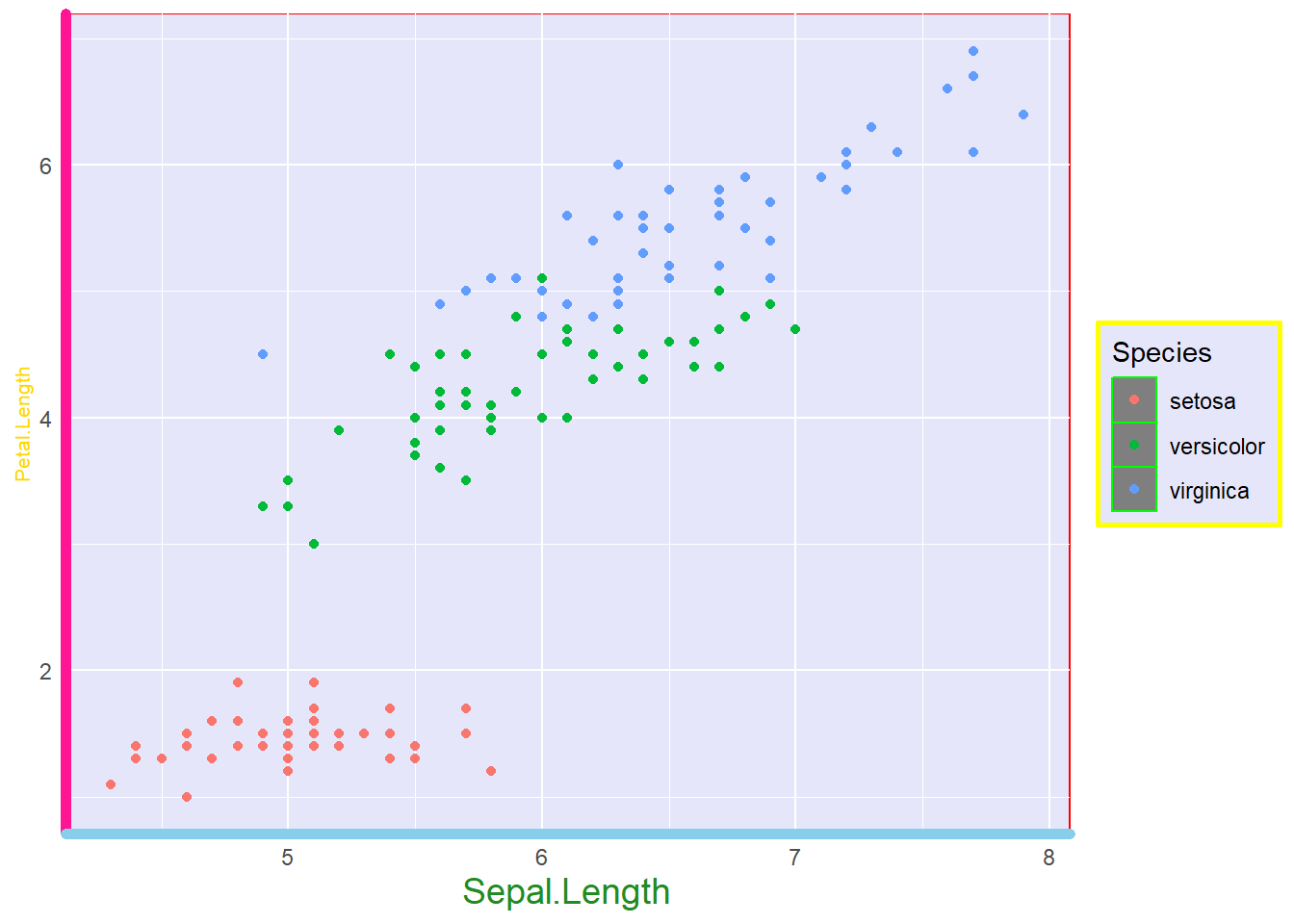# Axis lines

To change the axis lines and ticks (lines above each number on an axis) use the following.

Theme argument Description
axis.line = element_line(insert changes here) This will change both axes lines.
axis.line.x = element_line(insert changes here) This will change just the x axis.
axis.line.y = element_line(insert changes here) This will change just the y axis.
axis.ticks = element_line(insert changes here) Change both axes ticks. Use the .x or .y to change just one axis at a time.
axis.ticks.length = element_line(insert changes here) Change the length of the axes ticks.
axis.text = element_text(insert changes here) Change the text on the axes TICKS. Use .x or .y to change just one.
axis.title = element_text(insert changes here) Change the text on the axes LABELS/TITLES. Use .x or .y to change just one.
plot.title = element_text(insert changes here) Change the plot title.

Just use the colour and size arguments where appropriate. I am going to add these changes as a separate `theme()` command, but they can be added in the same command as last time.

``````iris.scatter + theme(panel.background = element_rect(fill="lavender", colour="red"), legend.background = element_rect(fill="lavender", colour="yellow", size=1), legend.key = element_rect(fill = "gray50", colour = "green", size = 0.5)) +
theme(axis.line.x = element_line(colour = "skyblue", size=2), axis.line.y = element_line(colour="deeppink", size = 2), axis.title.x = element_text(colour="forestgreen", size=14), axis.title.y = element_text(colour = "gold", size=8), axis.ticks = element_blank())``````Beautiful, isn’t it?

Now you may have notice the size command acts differently for line and text. For line it is based on a multiplier of the original. So a 2 will be two times its normal size. Element_text() has size as a font size. So 2 would be tiny and equivalent to 2pt font. Alternatively, you can use size = rel(number) to scale the text relative to base R’s plotting size.